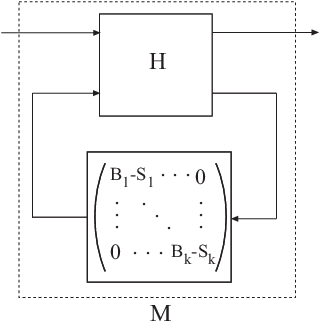getLFTModel

Decompose generalized LTI model

Syntax

[H,B,S] = getLFTModel(M)

Description

[H,B,S] = getLFTModel(M) extracts the components H, B, and S that make up the Generalized matrix or Generalized LTI model M. The model M decomposes into H, B, and S. These components are related to M as shown in the following illustration.The cell array B contains the Control Design Blocks of M. The component H is a numeric matrix, ss model, or frd model that describes the fixed portion of M and the interconnections between the blocks of B. The matrix S = blkdiag(S1,...,Sk) contains numerical offsets that ensure that the interconnection is well-defined when the current (nominal) value of M is finite.

You can recombine H, B, and S into M using lft, as follows:

M = lft(H,blkdiag(B{:})-S);

Input Arguments

 M Generalized LTI model (genss or genfrd) or Generalized matrix (genmat).

Output Arguments

 H Matrix, ss model, or frd model describing the numeric portion of M and how it the numeric portion is connected to the Control Design Blocks of M. B Cell array of Control Design Blocks (for example, realp or tunableSS) of M. S Matrix of offset values. The software might introduce offsets when you build a Generalized model to ensure that H is finite when the current (nominal) value of M is finite.